Electron. J. Diff. Eqns., Vol. 2000(2000), No. 67, pp. 1-22.

### Singular solutions of doubly singular parabolic equations with absorption Yuanwei Qi & Mingxin Wang

Abstract:
In this paper we study a doubly singular parabolic equation with absorption,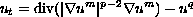with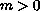,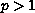,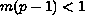, and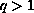. We give a complete classification of solutions, which we call singular, that are non-negative, non-trivial, continuous in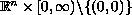, and satisfy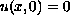for all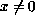. Applications of similar but simpler equations show that these solutions are very important in the study of intermediate asymptotic behavior of general solutions.

Submitted July 15, 2000. Published November 8, 2000.
Math Subject Classifications: 35K65, 35K15.
Key Words: doubly singular parabolic equation, absorption, singular solutions.

Show me the PDF file (223K), TEX file, and other files for this article.

Yuanwei Qi
Department of Mathematics
Hong Kong University of Science & Technology
Hong Kong,
email: maqi@uxmail.ust.hk

Mingxin Wang
Department of Applied Mathematics, Southeast University,
Nanjing 210018, P. R. China
email: mxwang@seu.edu.cn